# PSAT Math : How to find the probability of an outcome

## Example Questions

### Example Question #81 : Probability

We toss a fair 6-sided die twice. What is the probability that the first toss is bigger than the second toss.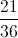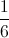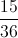Explanation:

If the first toss is a 1, it can not be bigger than any of the numbers on the second toss. If first toss is a 2, then it can be bigger than 1. If the first toss is 3, it can be bigger than 1 or 2. With this pattern, if the first toss is a 6, it can be bigger than any of the 5 numbers on the second toss.

Therefore, we get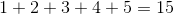ways to have the first toss being bigger than the second toss. The total number of combinations is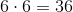.

So we have a probablility of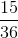.

### Example Question #91 : How To Find The Probability Of An Outcome

We randomly pick two numbers from positive integers. What is the probability that their sum is odd given that their product is even?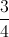Explanation:

There are four combinations total, being: {(odd,odd), (odd, even), (even, odd), (even, even)}

Given that their product is even, we only have the set {(odd,even),(even,odd),(even, even)}

The probability that their sum is odd from the set {(odd,even),(even,odd),(even, even)} isbecause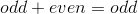and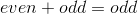.

### Example Question #81 : How To Find The Probability Of An Outcome

Bill rolls three 6-sided dice at once.  The first die comes up as a 5.  What is the probability that the total of all three dice will not be a prime number?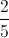Explanation:

The first die comes up as a 5.  We are essentially asking about outcomes of two dice, plus 5.  As a first step, we should be sure we know the primes we need to exempt.  The smallest sum we can have is if both of the other dice roll 1, giving us a 7.  7 is a prime number, so will will exempt this number. The largest number we can roll is both rolling 6, giving us a total of 17 (also a prime).  All the primes we will need to remove are then: 7,11,13 and 17.

We have two approaches now.  We can find the odds of rolling a number besides those above directly, or we can take the odds of getting any of the above 4, and then subtracting this value from 1.

The latter method is faster, but both use the same method.  Firstly, we'll subtract 5 from all the numbers: 2,6,8,12.

The odds of getting a 2 on two dice is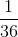, since the only roll that will work is two 1s and there are a total of 36 possible rolls (6 choices per die).

Similarly, the odds of getting a 12 issince it requires two 6's.

For 6, you can roll 1-5, 2-4, 3-3, 4-2, 5-1.  This gives us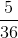odds of getting a 6.  Similarly, an 8 can be achieved by rolling 6-2, 5-3, 4-4, 3-5, 2-6.

Our total odds of rolling any of these 4 is thus: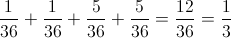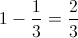### Example Question #1531 : Psat Mathematics

Angela has 20 red marbles, 8 yellow marbles, and 24 blue marbles.  If she

randomly chooses one marble from this set, what is the probablity that the

chosen marble is not blue?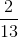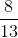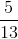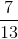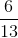Explanation: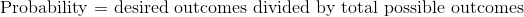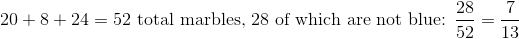### Example Question #211 : Data Analysis

A penny and a nickel are altered so that the penny comes up heads 54% of time when tossed and the nickel comes up heads 46% of the time when tossed. Both coins are tossed; what is the probability, to the nearest whole percent, that one comes up heads and the other comes up tails?

None of the other choices gives the correct answer.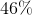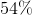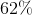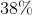None of the other choices gives the correct answer.

Explanation:

The penny will come up heads with probability 0.54 and tails with probability 0.46. The nickel will come up heads with probability 0.46 and probability 0.54.

The outcomes of the tosses of the penny and the nickel are independent, so the probabilities can be multiplied. The probability of the penny being heads and the nickel being tails is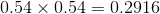. The probability of the reverse happening is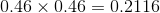.

These are disjoint, so add these probabilities; the probability that one head and one tail will come up will be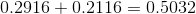, or 50.32 %. This rounds to 50%, which is not among the choices.

### Example Question #91 : How To Find The Probability Of An Outcome

A penny is altered so that the odds are 6 to 5 against it coming up tails when tossed; a nickel is altered so that the odds are 3 to 2 against it coming up tails when tossed. If both coins are tossed, what are the odds of there being two heads or two tails?

28 to 27 in favor

Even

9 to 5 against

28 to 27 against

9 to 5 in favor

28 to 27 in favor

Explanation:

6 to 5 odds in favor of heads is equal to a probability of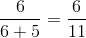, which is the probability that the penny will come up heads. The probability that the penny will come up tails is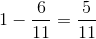.

Similarly, 3 to 2 odds in favor of heads is equal to a probability of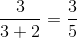, which is the probability that the nickel will come up heads. The probablity that the nickel will come up tails is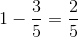.

The outcomes of the tosses of the penny and the nickel are independent, so the probabilities can be multiplied.

The probability of the penny and the nickel coming up heads is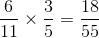.

The probability of the penny and the nickel coming up tails is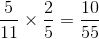.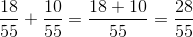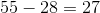, making the odds 28 to 27 in favor of both coins coming up the same.

### Example Question #91 : How To Find The Probability Of An Outcome

There are 78 marbles in a bag. If there are 2 times as many red marbles as blue, and 4 times as many blue marbles as green, what is the probability of choosing a blue marble on the first pick?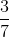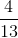Explanation:

Letrepresent the number of red marbles in the bag,represent the number of blue marbles, and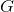represent the number of green.

We know the total number of marbles is 78: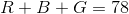We know there are 2 times as many red marbles as blue: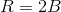And we know there are 4 times as many blue as green: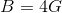Using substitution, we can see there are 8 times as many red marbles as green: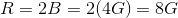Now, rewrite the original equation in terms of: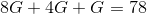Solve for.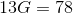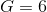There are 6 green marbles in the bag. Using this number, calculate the number of blue marbles in the bag: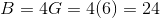There are 24 blue marbles in the bag. In order to find the probability of choosing a blue marble on the first pick, take the number of blue marbles in the bag and set it against the total number of marbles: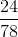This reduces to:The probability of choosing a blue marble on the first pick is.

### Example Question #91 : Probability

All of Jean's brothers have red hair.

If the statement above is true, then which of the following CANNOT be true?

If George does not have red hair, then he is Jean's brother.

If Winston does not have red hair, then he is not Jean's brother.

If Paul is not Jean's brother, then he has red hair.

If Eddie is Jean's brother, then he does not have red hair.

If Ron has red hair, then he is Jean's brother.

If Eddie is Jean's brother, then he does not have red hair.

Explanation:

Here we have a logic statement:

If A (Jean's brother), then B (red hair).

"If Ron has red hair, then he is Jean's brother" states "If B, then A" - we do not know whether or not this is true.

"If Winston does not have red hair, then he is not Jean's brother" states "If not B, then not A" - this has to be true.

"If Paul is not Jean's brother, then he has red hair" states "If not A, then B." We do not know whether or not this is true.

"If Eddie is Jean's brother, then he does not have red hair" states "If A, then not B" - we know this cannot be true.

### Example Question #92 : How To Find The Probability Of An Outcome

There are 10 balls in a lottery machine, each labeled with a number from 0 to 9. Each ball has a different number, and when one ball is selected from the machine, it is not replaced.  The machine ejects three balls that will form a three-digit winning lottery number. What is the probability that your one lottery ticket, with one three-digit number, will win?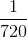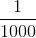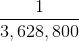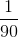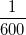Explanation:

First, find the number of possible three digit numbers that can be created from the lottery machine. Because the order that the numbers comes out matters (since the number 345 is different than 543), you should use the permutations formula: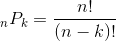Because you are choosing 3 balls out of 10, n=10 and k=3 so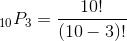This reduces to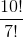which equals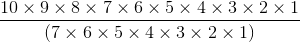You can then cancel out most of the numbers, leaving only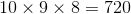Since you have only one three digit number on your lottery ticket, your probability of having the winning number is.

Note: Because the balls are not replaced, this question is really asking "How many 3-digit numbers are there in which no integer appears more than once?"

### Example Question #251 : Data Analysis

Ben only goes to the park when it is sunny.

If the above statement is true, which of the following is also true?

If Ben is not at the park, it is not sunny.

If it is sunny, Ben is at the park.

If it is not sunny, Ben is not at the park.

If it is rainy, Ben is at the park.